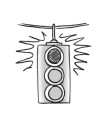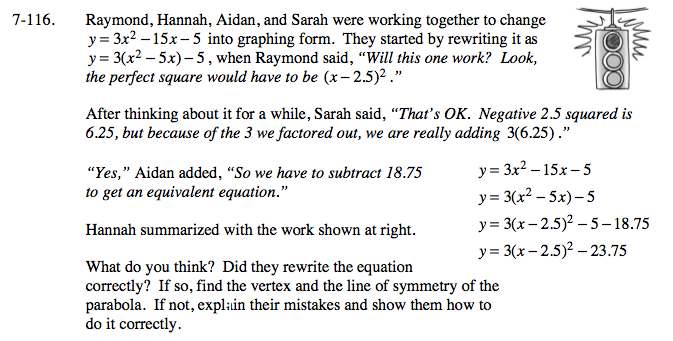Home > A2C > Chapter 7 > Lesson 7.2.2 > Problem7-116

7-116.
1. Raymond, Hannah, Aidan, and Sarah were working together to change y = 3x2 − 15x − 5 into graphing form. They started by rewriting it as y = 3(x2 − 5x) − 5, when Raymond said,Will this one work? Look, the perfect square would have to be (x − 2.5)2.

After thinking about it for a while, Sarah said, “that's OK. Negative 2.5 squared is 6.25, but because of the 3 we factored out, we are really adding 3(6.25).”

2. Yes,” Aidan added, “So we have to subtract 18.75 to get an equivalent equation.”

3. Hannah summarized with the work shown below. What do you think? Did they rewrite the equation correctly? If so, find the vertex and the line of symmetry of the parabola. If not, explain their mistakes and show them how to do it correctly. Homework Help ✎

y = 3x2 −15x − 5
y = 3(x2 − 5x) − 5
y = 3(x − 2.5)2 − 5 −18.75
y = 3(x − 2.5)2 − 23.75You can tell if the two equations are equivalent by working backwards from their equation.